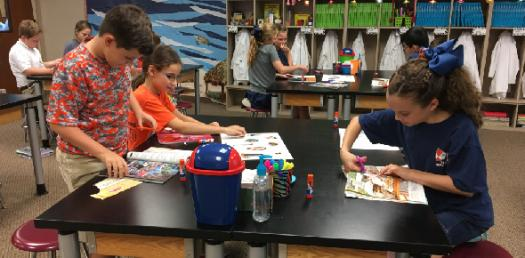# Trivia: 5th Grade Science Benchmark Test

23 Questions | Total Attempts: 152SettingsThere are certain things that one would be expected to have a good idea of especially when they get to the fifth grade. This is like understanding scientific processes and explaining how they work. Feel like you know them and can tackle any exam that is a result of it? Take up the quiz below and get to find out!

• 1.
Matter that is liquid tends to be more dense than matter that is
• A.

Solid

• B.

Magnetic

• C.

Electrical

• D.

Gaseous

• 2.
The temperature at which a liquid becomes a gas is the _____________ point.
• A.

Freezing

• B.

Boiling

• C.

Condensation

• D.

Melting

• 3.
A good conductor of heat energy is a
• A.

Wooden spoon.

• B.

Glass plate.

• C.

Metal spoon.

• D.

Plastic spoon.

• 4.
Which would be true if you took an object to the moon?
• A.

The object would have less mass.

• B.

The object would have more mass.

• C.

The object would weigh less.

• D.

The objecct would weigh more.

• 5.
One approach to identifying something unknown is to use a _______________.
• A.

Dichotomous key

• B.

Lightstick

• C.

Liquid layer

• D.

Control

• 6.
Milliliters are the unit of measure for what property of matter?
• A.

Density

• B.

Volume

• C.

Magnetism

• D.

Mass

• 7.
An example of a physical change is
• A.

Water evaporating.

• B.

Metal rusting.

• C.

A candle burning.

• D.

A cake baking.

• 8.
When a peeled banana turns black, it is a ___________________.
• A.

Chemical change

• B.

Physical change

• C.

Change of state

• D.

Thermal change

• 9.
An example of a chemical change is  ________________________.
• A.

A magnet sticking to a refridgerator

• B.

Dew disappearing from the grass

• C.

Mashing potatoes

• D.

Frying a hamburger

• 10.
Gravity is a
• A.

Velocity.

• B.

Speed.

• C.

Force.

• D.

Weight.

• 11.
Which describes how velocity changes with time?
• A.

Acceleration

• B.

Speed

• C.

Force

• D.

Weight

• 12.
A person in a head-on car collision who is not wearing a seat belt continues to move forward at the original speed of the car because of _______________.
• A.

Friction

• B.

Inertia

• C.

Gravity

• D.

Weight

• 13.
A rock rolling down a hill has
• A.

Kinectic energy.

• B.

Potential energy.

• C.

No energy.

• D.

Electrical energy.

• 14.
Force is measured in which units?
• A.

Kilograms

• B.

Degrees

• C.

Newtons

• D.

M/s2

• 15.
Energy occurs in ___________________.
• A.

Only one form

• B.

Only two forms

• C.

Only three forms

• D.

Many forms

• 16.
What are the two main types of energy that relate to motion?
• A.

Conduction and convection

• B.

Solar and thermal

• C.

Potential and kinetic

• D.

• 17.
As the temperature of a material increases, the average ____________ of its particles also increases.
• A.

Specific heat

• B.

Kinetic energy

• C.

Mass

• D.

Potential energy

• 18.
A rock sitting on top of a cliff has _____________________.
• A.

Kinetic energy

• B.

No energy

• C.

Potential energy

• D.

• 19.
What is the freezing point of water on the Celsius temperature scale?
• A.

0 Degree Celsius

• B.

-100 Degree Celsius

• C.

100 Degree Celsius

• D.

273 Degree Celsius

• 20.
Which one of the following statements describes the flow of heat?
• A.

Energy moves from a warmer object to a cooler object.

• B.

Energy moves from a cooler object to a warmer object.

• C.

Energy moves only between two warm objects.

• D.

Energy moves only between two cold objects.

• 21.
You hypothesize that sugar is more soluble in water than salt. In conducting your experiment, which is not a variable that you need to control?
• A.

The amount of water used

• B.

The temperature of the water

• C.

The amount of each solute used

• D.

The shape of the container you mix the soloution in

• 22.
If a ball is rolling and there is no force acting on the ball, then
• A.

The ball will stop.

• B.

The ball will roll forever.

• C.

The ball will speed up.

• D.

The ball will slow down

• 23.
When an object is at rest, what is its speed?
• A.

2 m/s

• B.

3 m/s

• C.

1 m/s

• D.

0 m/s

Related TopicsBack to top